Question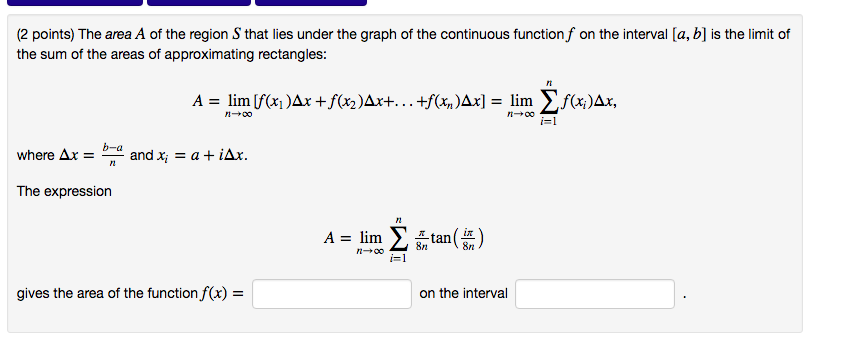(2 points) The area $$A$$ of the region $$S$$ that lies under the graph of the continuous function $$f$$ on the interval $$[a, b]$$ is the limit of the sum of the areas of approximating rectangles:

$$A=\lim _{n \rightarrow \infty}\left[f\left(x_{1}\right) \Delta x+f\left(x_{2}\right) \Delta x+\ldots+f\left(x_{n}\right) \Delta x\right]=\lim _{n \rightarrow \infty} \sum_{i=1}^{n} f\left(x_{i}\right) \Delta x$$

where $$\Delta x=\frac{b-a}{n}$$ and $$x_{i}=a+i \Delta x$$.

The expression

$$A=\lim _{n \rightarrow \infty} \sum_{i=1}^{n} \frac{\pi}{8 n} \tan \left(\frac{i \pi}{8 n}\right)$$

gives the area of the function $$f(x)=$$ on the interval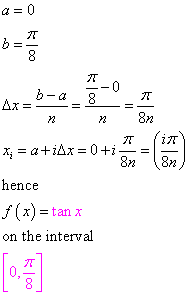#### Earn Coins

Coins can be redeemed for fabulous gifts.

Similar Homework Help Questions
• ### find an expression for the area of the region under the graphf(x)=x^4 on the interval...

find an expression for the area of the region under the graph f(x)=x^4 on the interval [1,7]. use right-Hand endpoints as sample points choices1. area $$=\lim _{n \rightarrow \infty} \sum_{i=1}^{n}\left(1+\frac{7 i}{n}\right)^{4} \frac{7}{n}$$2. area $$=\lim _{n \rightarrow \infty} \sum_{i=1}^{n}\left(1+\frac{9 i}{n}\right)^{4} \frac{6}{n}$$3. area $$=\lim _{n \rightarrow \infty} \sum_{i=1}^{n}\left(1+\frac{6 i}{n}\right)^{4} \frac{6}{n}$$4. area $$=\lim _{n \rightarrow \infty} \sum_{i=1}^{n}\left(1+\frac{7 i}{n}\right)^{4} \frac{6}{n}$$5. area $$=\lim _{n \rightarrow \infty} \sum_{i=1}^{n}\left(1+\frac{6 i}{n}\right)^{4} \frac{7}{n}$$6. area $$=\lim _{n \rightarrow \infty} \sum_{i=1}^{n}\left(1+\frac{9 i}{n}\right)^{4} \frac{7}{n}$$

• ### (1 point) Definition: The AREA A of the region that lies under the graph of the...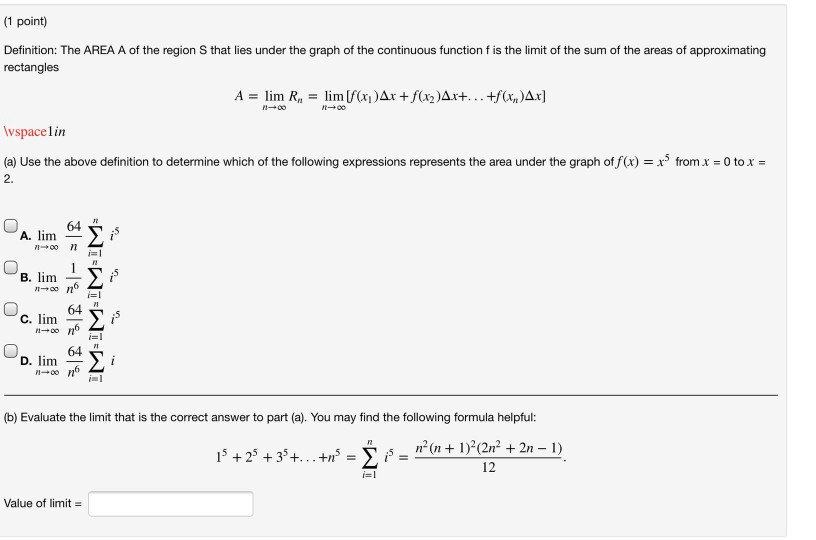(1 point) Definition: The AREA A of the region that lies under the graph of the continuous function f is the limit of the sum of the areas of approximating rectangles A = lim R, = lim [f(x)Ar + f(x2)Ax+... +f(x,y)Ax] 100 Wspacelin (a) Use the above definition to determine which of the following expressions represents the area under the graph of f(x) = x3 from x = 0 to x = 2. 64 A. lim 7100 11 i= B....

• ### -/2 POINTS SESSCALCET2 5.1.503.XP. The area A of the region that lies under the graph of...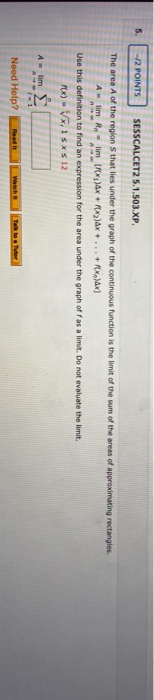-/2 POINTS SESSCALCET2 5.1.503.XP. The area A of the region that lies under the graph of the continuous function is the limit of the sum of the areas of approximating rectangles. lim Rn limf ax + 2)Ax + ... + X)x] Use this definition to find an expression for the area under the graph off as a limit. Do not evaluate the limit. FX) - VX,15* \$ 12 A lim Need Help? Talk to Tutor

• ### The area A of the region S that les under the graph of the continuous fun the areas of approximat...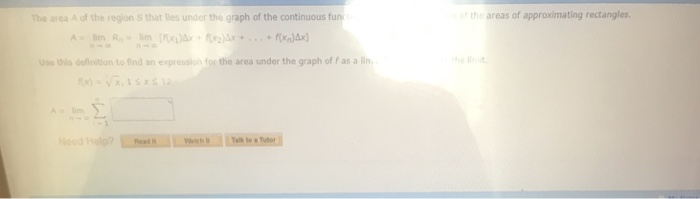The area A of the region S that les under the graph of the continuous fun the areas of approximating rectangles sthis deinition to find an expression for the area under the graph of f as a The area A of the region S that lies under the graph of the continuous function is the limit of the sum of the areas of approximating rectangles Use this definition to find an expression for the area under the graph of f...

• ### ssignment6: Problem 9 Previous Problem Problem List Next Problem (1 point) The area A of the...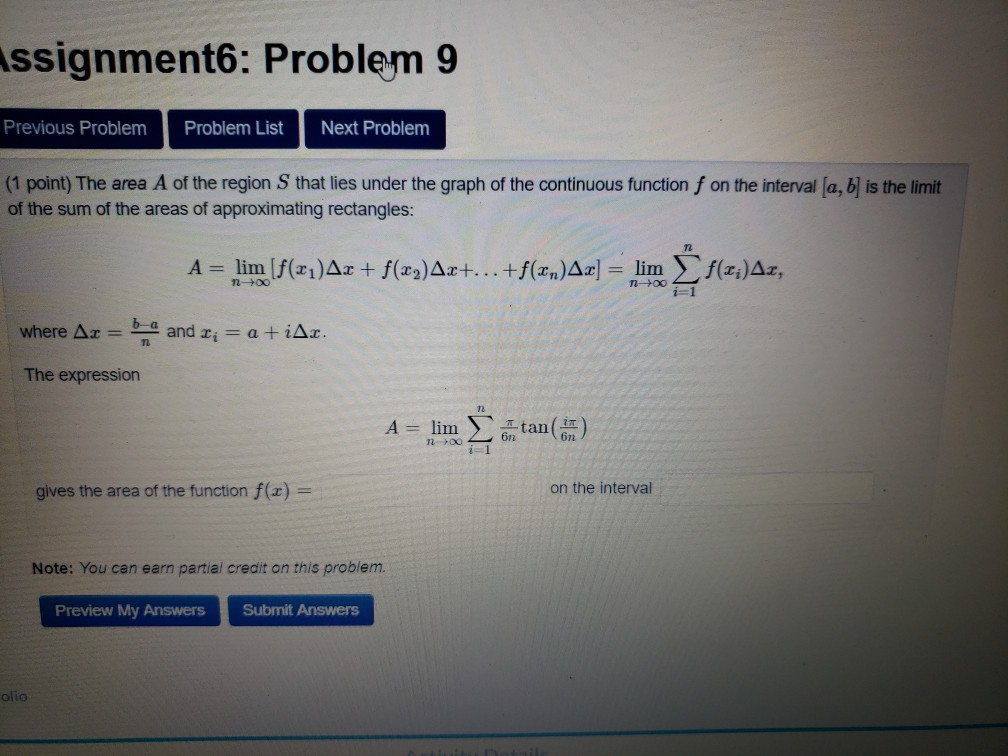ssignment6: Problem 9 Previous Problem Problem List Next Problem (1 point) The area A of the region Sthat lies under the graph of the continuous function f on the interval (a, b) is the limit of the sum of the areas of approximating rectangles: A = lim (f(21)Ar + f(x2)Ax+...+f(xn)Ax] = lim f(x;)Az, n-> ng i=1 where Ax = b and Ti = a +iAr. The expression A = lim Itan(n) 7200 6n2 gives the area of the function f(x)...

• ### Frequency-domain sampling using Fourier series

Frequency-domain sampling. Consider the following discrete-time signal$$x(n)= \begin{cases}a^{|n|}, & |n| \leq L \\ 0, & |n|>L\end{cases}$$where $$a=0.95$$ and $$L=10$$.(a) Compute and plot the signal $$x(n)$$.(b) Show that$$X(\omega)=\sum_{n=-\infty}^{\infty} x(n) e^{-j \omega n}=x(0)+2 \sum_{n-1}^{L} x(n) \cos \omega n$$Plot $$X(\omega)$$ by computing it at $$\omega=\pi k / 100, k=0,1, \ldots, 100$$.(c) Compute$$c_{k}=\frac{1}{N} X\left(\frac{2 \pi}{N} K\right), \quad k=0,1, \ldots, N-1$$for $$N=30$$.(d) Determine and plot the signal$$\tilde{x}(n)=\sum_{k=0}^{N-1} c k e^{j(2 \pi / N) k n}$$What is the...

• ### Consider a discrete time signal

Consider a discrete time signal $$y[n]=\frac{\sin (\pi n / 5)}{\pi n}+\cos (\pi n / 10) .$$ Let's build the continuous time representation of $$y[n]$$ using $$T_{s}=1 / 10$$ as follows:$$y_{\delta}(t)=\sum_{n=-\infty}^{\infty} y[n] \delta\left(t-n T_{s}\right)=\sum_{n=-\infty}^{\infty} y[n] \delta(t-n / 10)$$Choose the right expression for $$Y_{\delta}(j \omega)$$.(Hint: You can first sketch $$Y\left(e^{j \Omega}\right)$$. Then, you can obtain the sketch of $$Y_{\delta}(j \omega)$$ from $$Y\left(e^{j \Omega}\right)$$. Note that in the earlier lecture on sampling, we derived that $$Y_{\delta}(j \omega)=\left.Y\left(e^{j \Omega}\right)\right|_{\Omega=\omega T_{x}}=Y\left(e^{j \omega T_{\nu}}\right)$$.In...

• ### send help for these 4 questions, please show steps Definition: The AREA A of the region...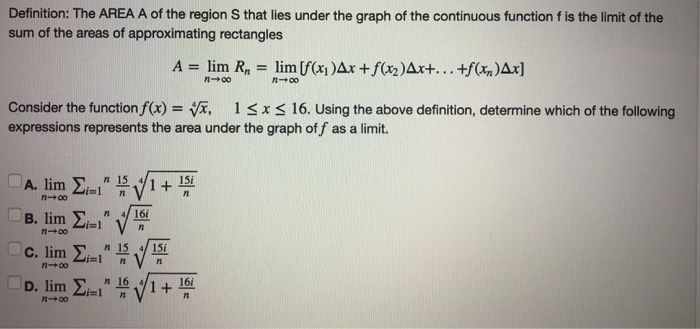send help for these 4 questions, please show steps Definition: The AREA A of the region S that lies under the graph of the continuous function f is the limit of the sum of the areas of approximating rectangles A = lim R, = lim [f(x)Ax +f(x2)Ax+...+f(x)Ax] - 00 Consider the function f(x) = x, 13x < 16. Using the above definition, determine which of the following expressions represents the area under the graph off as a limit. A. lim...